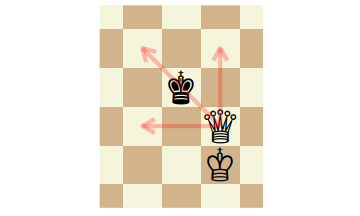I cringe when I hear the term "Twelve Dimensional Chess" used as a metaphor. Certainly Twelve Dimensional Chess would be hard to visualize, and would present far more possible moves than regular two dimensional chess. However, high dimensional Chess suffers from a Curse of Dimensionality as the number of squares grows so quickly that play becomes uninteresting. In fact, I suspect that strategies exist which effectively guarantee a draw in sufficiently high dimensions.

Consider a Queen attacking a King in our stupid old Two Dimensional Chess. The Queen can cover or attack seven of the nine squares available to the King, as shown below:If the two remaining squares are occupied, or do not exist because the King is against the boundary, the Queen can give checkmate.

However, in higher dimensional Chess, the Queen attacks a far smaller proportion of the squares adjacent to a King. In dimension $$d$$ the number attacked or covered is $$2^{d+1} - 1$$. For $$d=12$$, the number of squares attacked is $$8191$$. However, the King has $$3^{12}=531441$$ squares in his neighborhood when not against the boundary. The queen covers only about $$1.54\%$$ of these squares, so you would need $$65$$ Queens to give checkmate. A non-losing strategy seems to be:

Move your King away from the boundary on your first move, and keep away from the boundary. Your opponent cannot promote enough pawns to give checkmate.

Actually, I do not know the rules of high dimensional Chess, and I have assumed the players start with eight pieces and eight pawns. Maybe the number of pawns is linear, or even exponential in the dimension. Even so, it will take over $$300$$ moves to promote the $$64$$ pawns to Queens. Moreover, assuming one's opponent could muster such an army without any losses, assembling such a large number of Queens in place to achieve checkmate might be tricky. Each Queen attacks at most $$1.86$$ million squares. Again, this would be hard to visualize during play, but there are $$2.2$$ billion internal squares (i.e. those not touching a boundary), and some $$68.7$$ billion in total. Which means that even if your opponent has dozens of Queens on the board, each Queen can attack only a small fraction of the available squares. You could move your King largely at random without coming under attack.

So "Twelve Dimensional Chess" as a metaphor for a situation requiring great foresight or strategy in the face of many possible decision is flawed. Instead, it is a more apt metaphor for a very lonely random walk punctuated by infrequent interactions with others you can easily dodge.